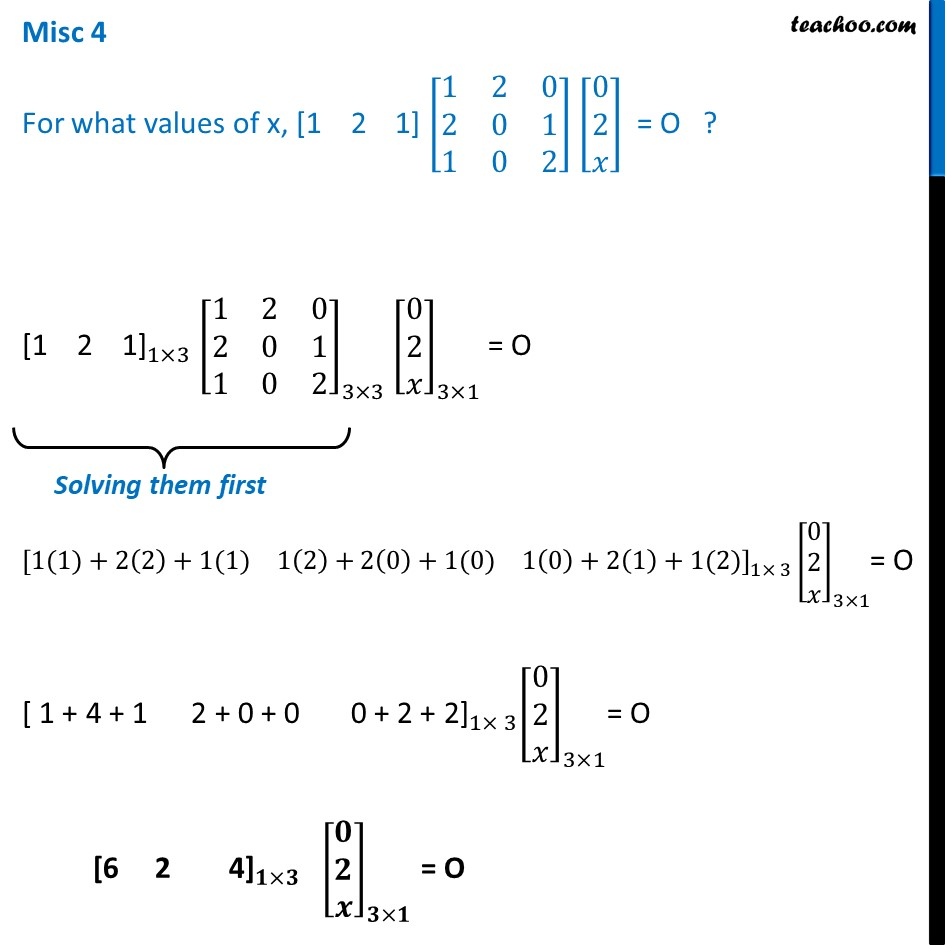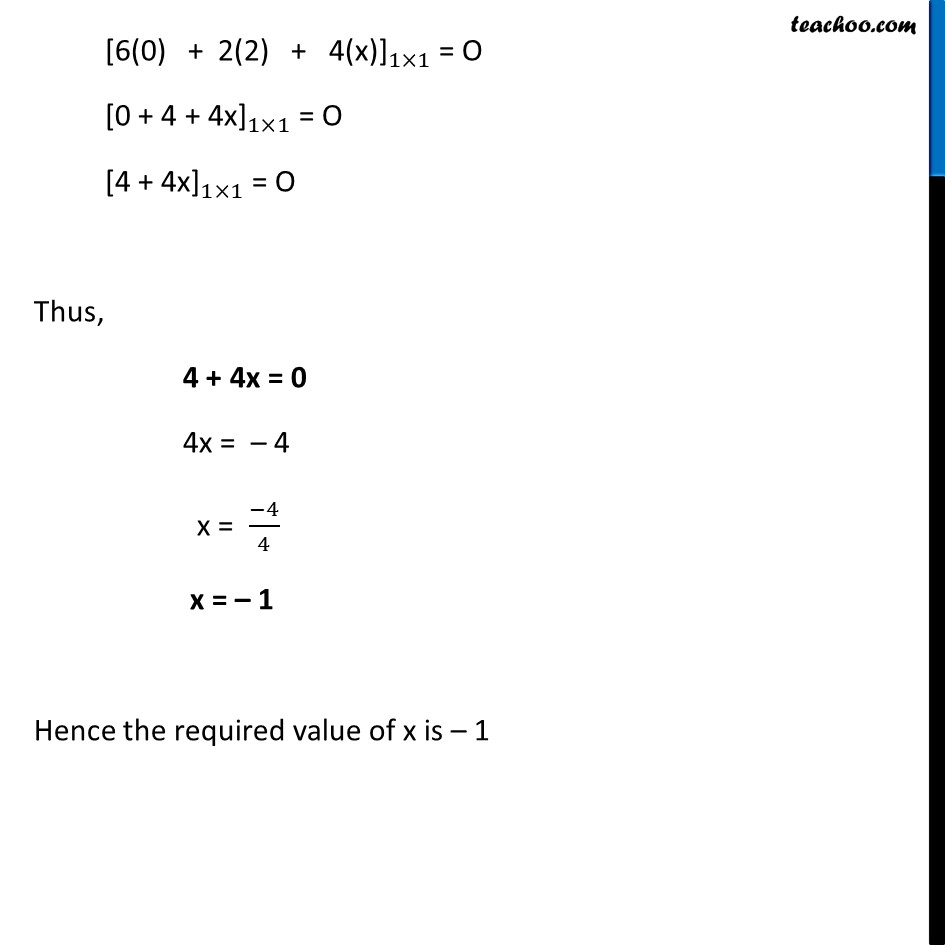Finding unknown - Element

Chapter 3 Class 12 Matrices
Concept wiseLearn in your speed, with individual attention - Teachoo Maths 1-on-1 Class

### Transcript

Misc 4 For what values of x, [1 2 1] [■8(1&2&0@2&0&1@1&0&2)] [■8(0@2@𝑥)] = O ? 〖"[1 2 1]" 〗_(1×3) [■8(1&2&0@2&0&1@1&0&2)]_(3×3) [■8(0@2@𝑥)]_(3×1) = O [■8(1(1)+2(2)+1(1)&1(2)+2(0)+1(0)&1(0)+2(1)+1(2))]_(1× 3) [■8(0@2@𝑥)]_(3×1)= O 〖"[ 1 + 4 + 1 2 + 0 + 0 0 + 2 + 2]" 〗_(1× 3) [■8(0@2@𝑥)]_(3×1)= O 〖"[6 2 4]" 〗_(𝟏×𝟑) [■8(𝟎@𝟐@𝒙)]_(𝟑×𝟏) = O 〖"[6(0) + 2(2) + 4(x)]" 〗_(1×1) = O 〖"[0 + 4 + 4x]" 〗_(1×1) = O 〖"[4 + 4x]" 〗_(1×1) = O Thus, 4 + 4x = 0 4x = – 4 x = (−4)/4 x = – 1 Hence the required value of x is – 1